Next: Sudden Perturbations Up: Time-Dependent Perturbation Theory Previous: Nuclear Magnetic Resonance

# Dyson Series

Let us now try to find approximate solutions to Equation (8.11) for a general system. It is convenient to work in terms of the time evolution operator,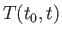, which is defined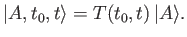(8.37)

(See Section 3.2.) As before,is the state ket of the system at time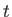, given that the state ket at the initial time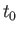is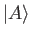. It is easily seen that the time evolution operator satisfies the differential equation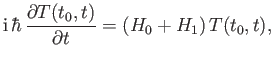(8.38)

subject to the initial condition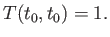(8.39)

(See Section 3.2.)

In the absence of the external perturbation, the time evolution operator reduces to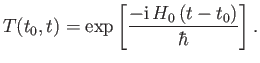(8.40)

Let us switch on the perturbation, and look for a solution of the form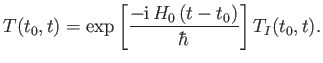(8.41)

It is readily demonstrated that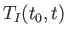satisfies the differential equation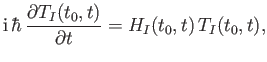(8.42)

where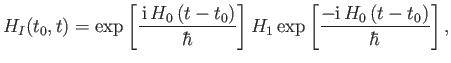(8.43)

subject to the initial condition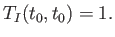(8.44)

(See Exercise 3.) Note that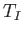specifies that component of the time evolution operator that is generated by the time-dependent perturbation. Thus, we would expectto contain all of the information regarding transitions between different eigenstates of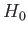caused by the perturbation.

Suppose that the system starts off at timein the eigenstate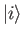of the unperturbed Hamiltonian. The subsequent evolution of the state ket is given by Equation (8.6),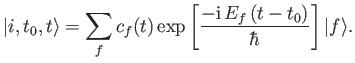(8.45)

However, we also have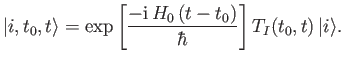(8.46)

It follows that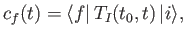(8.47)

where use has been made of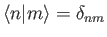. Thus, the probability that the system is found in some final state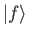at time, given that it was definitely in the initial stateat time, is simply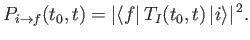(8.48)

This quantity is usually termed the transition probability between statesand.

Note that the differential equation (8.45), plus the initial condition (8.47), are equivalent to the following integral equation: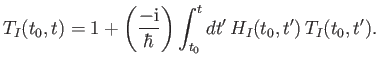(8.49)

We can obtain an approximate solution to this equation via iteration: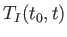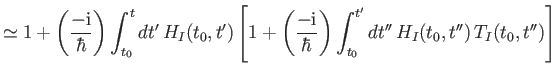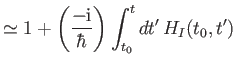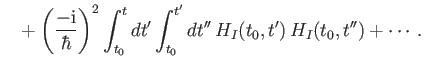(8.50)

This expansion is known as the Dyson series .

Let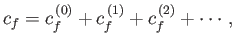(8.51)

where the superscript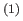refers to a first-order term in the expansion, et cetera. It follows from Equations (8.50) and (8.53) that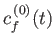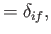(8.52)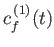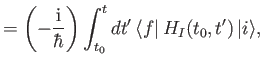(8.53)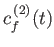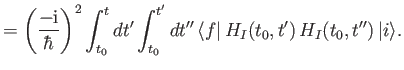(8.54)

These expressions simplify to give(8.55)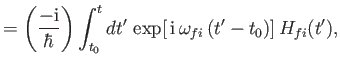(8.56)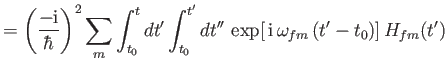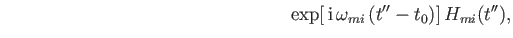(8.57)

where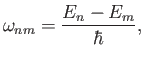(8.58)

and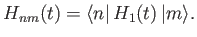(8.59)

Here, use has been made of the completeness relation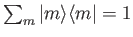, where the sum is over all energy eigenstates. The transition probability between states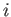and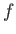is simply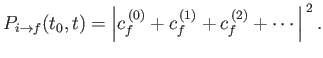(8.60)

According to the previous analysis, there is no possibility of a transition between the initial state,, and the final state,, (where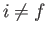) to zeroth order (i.e., in the absence of the perturbation). To first order, the transition probability is proportional to the time integral of the matrix element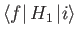, weighted by an oscillatory phase-factor. Thus, if the matrix element is zero then there is no chance of a first-order transition between statesand. However, to second order, a transition between statesandis possible even when the matrix elementis zero.Next: Sudden Perturbations Up: Time-Dependent Perturbation Theory Previous: Nuclear Magnetic Resonance
Richard Fitzpatrick 2016-01-22